# Similarity Theory

The following article is from The Great Soviet Encyclopedia (1979). It might be outdated or ideologically biased.

## Similarity Theory

the science of the conditions under which physical phenomena are similar. It is related to dimensional analysis and provides the basis for physical modeling. Similarity theory establishes similarity criteria for different physical phenomena and studies the properties of the phenomena by means of these criteria.

Two physical phenomena, processes, or systems are similar if, at corresponding moments of time at corresponding points in space, the values of the variable quantities that characterize the state of one system are proportional to the corresponding quantities of the second system. The proportionality factor for each of the quantities is called the similarity factor.

Physical similarity is a generalization of the elementary and intuitively obvious concept of geometric similarity. In geometry, if corresponding geometrical elements of two figures or solids are in proportion, the figures are said to be similar. In physical similarity, the fields of the corresponding physical parameters of two systems are similar in space and time. For example, kinematic similarity is characterized by the similarity of the velocity fields of the two motions under consideration. In dynamic similarity, systems of different types of acting forces or force fields—such as gravity forces, pressure forces, and viscous forces —are similar. The mechanical similarity of, for example, two liquid or gas flows or two elastic systems presupposes geometric, kinematic, and dynamic similarity. Similarity between thermal processes exists when the corresponding temperature and heat-flow fields are similar. Finally, electrodynamic similarity occurs if the current, load, and power fields and the electromagnetic force fields are similar. The enumerated types of similarity are special cases of physical similarity.

The development of research on complex physical and physicochemical processes, including mechanical, thermal, and chemical phenomena, has been accompanied by the development of methods of similarity theory that can be applied to these processes. For example, similarity conditions have been established for such phenomena as the friction and abrasion processes undergone by machine parts and the kinetics of physicochemical transformations. Since the parameters characterizing similar phenomena are in proportion, any dimensionless combination of the parameters will have the same numerical value for similar phenomena. Dimensionless combinations formed from the parameters of the phenomena under consideration are called similarity criteria. Any combination of similarity criteria is also a similarity criterion of these physical phenomena.

Incomplete, or partial, similarity occurs if some, but not all, of the independent similarity criteria of the physical phenomena or systems being investigated are equal. This is the situation most often encountered in practice. When this situation occurs, it is essential that the unequal criteria have only an insignificant effect on the physical processes under consideration.

The dimensional physical parameters that form similarity criteria may take on markedly different values for similar systems. Only the dimensionless similarity criteria must be equal. This property of similar systems is the basis for modeling.

S. L. VISHNEVETSKII

A more rigorous presentation of the logical foundations of similarity theory follows. Let us assume that a description of given phenomena uses r independent fundamental units of measurement A1, A2, ….., Ar; for example, in absolute systems of units the fundamental units are the units of length L, mass M, and time T. The derived units of measurement have the form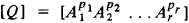Their dimension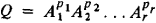is determined by the numerical exponents p1, p2, …, pr. Every quantity X of dimension [X] = [Q] is represented in the form X = xQ, where x is the numerical expression of X in the chosen system of fundamental quantities A1, A2, …. Ar.

Suppose we are studying a class S of phenomena such that each phenomenon is defined by assigning certain values of the system of quantities {Yα}. Two such phenomena S(1) and S(2) are said to be similar if the values of the quantities Yα(2) that characterize S(2) are obtained from the values of the corresponding quantities Yα(1) that characterize S(1) by the formulas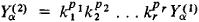where the similarity factors k1, k2, …, kr are constant and the exponents p1, p2, …, p, are determined by the dimension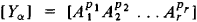of the quantities Yα.

Let us assume that part of the system of quantities {Yα} has been singled out and that this part forms a system {Xβ} of defining parameters of the following type: the numerical values yαα of any quantity Yα is a function Yα = f{xβ} of the numerical values xβ of the quantities Xβ, and the form of the functional relationship fα remains invariant under any choice of the fundamental units of measurement A1, A2, …, Ar Under this assumption, the fundamental principle of similarity theory can be formulated in the following way. Two phenomena S(1) and S(2) are similar if and only if the values of any dimensionless combination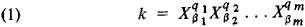of the defining parameters of S(1) and S(2) are equal: k(1) = k(2).

Any dimensionless expression k of the form of (1) is called a similarity criterion. By this definition, similarity criteria evidently include all dimensionless parameters and all ratios of the form

(2) k = Xβ1/Xβ2

where Xβ1, and 2 are parameters with the same dimension.

It follows that the equalities k(1) = k (2) must hold for dimensionless parameters and ratios of the form (2) if similarity is to occur. Such equalities may be called trivial. Ratios k of the form of (2) are often dropped when similarity criteria are listed. If the trivial conditions k(1) = k(2) are regarded as automatically satisfied, there are only s = nr’ independent nontrivial similarity conditions k(1) = k(2), where n is the number of different dimensions of the quantities of the system {Xβ} and r’ is the number of these n dimensions that are independent. Since r’r always, s ≤ nr.

Let us consider, for example, the steady flow around a rectangular plate located in a uniform unbounded stream of a viscous incompressible fluid whose velocity at infinity is parallel to the longitudinal side of the plate. The geometric picture of this flow is determined by (1) the plate length l, (2) the width b of the plate, (3) the flow velocity at infinity v, and (4) the coefficient of kinematic viscosity v. Since [b] = [l] and [v] = [vl], only two of the three dimensions of the defining parameters are independent; that is, r’ = 2 and s = nr’ = 3 –2 = 1. Thus, there is a single nontrivial similarity criterion, the Reynolds number Re = vl/v. In addition, there is a trivial similarity criterion, b/l. If the phenomena under investigation are studied by means of differential equations, the defining parameters occur as quantities in the initial and boundary conditions or as coefficients in the differential equations. When the equations are reduced to dimensionless form, only dimensionless coefficients remain in them; these coefficients are the similarity criteria.

For example, the equations of the steady-state motion of an incompressible viscous fluid are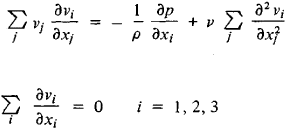where p is the fluid pressure, vi the velocity components, and are the Cartesian coordinates. These equations can be reduced to dimensionless form by the transformation xi = ξil,vi = ηi v, p = ξpv2. In the new variables ξi, ηi, and ξ, the equations have the form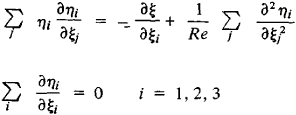A. N. KOLMOGOROV

Similarity theory has many practical applications. It makes possible preliminary qualitative theoretical analysis and permits the selection of a system of controlling dimensionless parameters for complex physical phenomena. It provides a basis for the correct formulation of experiments and the processing of experimental results. When combined with additional considerations drawn from experimentation or the equations describing a physical phenomenon, similarity theory can lead to important new results.

### REFERENCES

Sedov, L. I. Metodypodobiia i razmernosti v mekhanike, 7th ed. Moscow, 1972.
Eigenson, L. S. Modelirovanie. Moscow, 1952.
Venikov, V. A. Teoriia podobiia i modelirovanie primenitel’no k zadacham elektroenergetiki. Moscow, 1966.
Kirpichev, M. V. Teoriia podobiia, Moscow, 1953.
D’iakonov, G. K. Voprosy teorii podobiia v oblasti fiziko-khimicheskikh protsessov. Moscow-Leningrad, 1956.